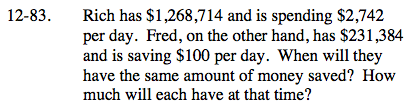### Home > CAAC > Chapter 12 > Lesson 12.4.2 > Problem12-83

12-83.Rich has $1,268,714 and is spending$2,742 per day. Fred, on the other hand, has $231,384 and is saving$100 per day. When will they have the same amount of money saved? How much will each have at that time? Homework Help ✎Create a system of equations to represent the problem.

Equation 1: y = −2742x + 1268714

Write the other equation and solve.